## Domain and Range of Functions

### Learning Outcomes

• Find the domain of a function defined by an equation.
• Write the domain and range using standard notations.
• Find domain and range from a graph.
• Give domain and range of Toolkit Functions.
• Graph piecewise-defined functions.

If you’re in the mood for a scary movie, you may want to check out one of the five most popular horror movies of all time—I am Legend, Hannibal, The Ring, The Grudge, and The Conjuring. Figure 1 shows the amount, in dollars, each of those movies grossed when they were released as well as the ticket sales for horror movies in general by year. Notice that we can use the data to create a function of the amount each movie earned or the total ticket sales for all horror movies by year. In creating various functions using the data, we can identify different independent and dependent variables, and we can analyze the data and the functions to determine the domain and range. In this section we will investigate methods for determining the domain and range of functions such as these.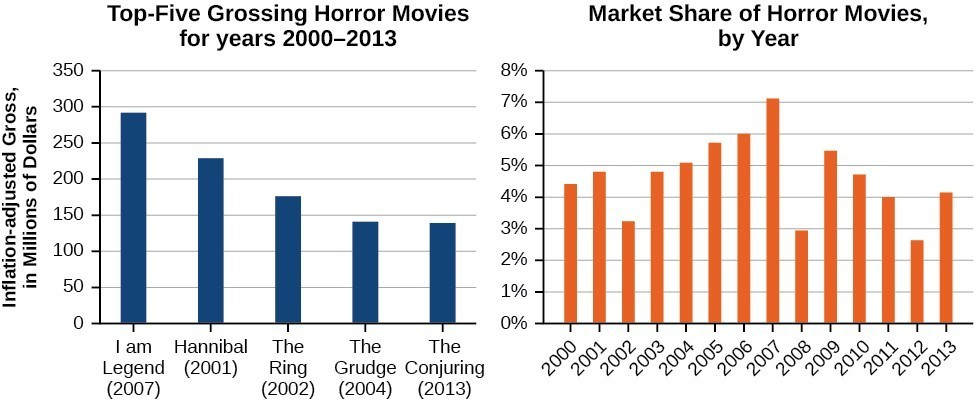Figure 1. Based on data compiled by www.the-numbers.com.

## Standard Notation for Defining Sets

There are several ways to define sets of numbers or mathematical objects. The reason we are introducing this here is because we often need to define the sets of numbers that make up the inputs and outputs of a function.

How we write sets that make up the domain and range of functions often depends on how the relation or function are defined or presented to us.  For example, we can use lists to describe the domain of functions that are given as sets of ordered pairs. If we are given an equation or graph, we might use inequalities or intervals to describe domain and range.

In this section, we will introduce the standard notation used to define sets, and give you a chance to practice writing sets in three ways, inequality notation, set-builder notation, and interval notation.

Consider the set $\left\{x|10\le x<30\right\}$, which describes the behavior of $x$ in set-builder notation. The braces $\{\}$ are read as “the set of,” and the vertical bar $|$ is read as “such that,” so we would read $\left\{x|10\le x<30\right\}$ as “the set of x-values such that 10 is less than or equal to $x$, and $x$ is less than 30.”

The table below compares inequality notation, set-builder notation, and interval notation.

Inequality Notation Set-builder Notation Interval Notation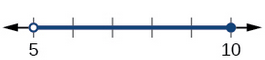$5<h\le10$ $\{h | 5 < h \le 10\}$ $(5,10]$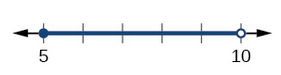$5\le h<10$ $\{h | 5 \le h < 10\}$ $[5,10)$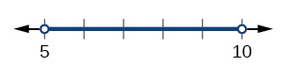$5<h<10$ $\{h | 5 < h < 10\}$ $(5,10)$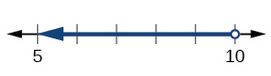$h<10$ $\{h | h < 10\}$ $(-\infty,10)$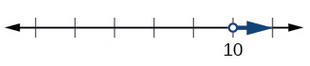$h>10$ $\{h | h > 10\}$ $(10,\infty)$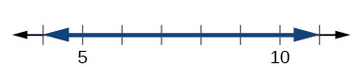All real numbers $\mathbf{R}$ $(−\infty,\infty)$

To combine two intervals using inequality notation or set-builder notation, we use the word “or.” As we saw in earlier examples, we use the union symbol, $\cup$, to combine two unconnected intervals. For example, the union of the sets $\left\{2,3,5\right\}$ and $\left\{4,6\right\}$ is the set $\left\{2,3,4,5,6\right\}$. It is the set of all elements that belong to one or the other (or both) of the original two sets. For sets with a finite number of elements like these, the elements do not have to be listed in ascending order of numerical value. If the original two sets have some elements in common, those elements should be listed only once in the union set. For sets of real numbers on intervals, another example of a union is

$\left\{x|\text{ }|x|\ge 3\right\}=\left(-\infty ,-3\right]\cup \left[3,\infty \right)$

This video describes how to use interval notation to describe a set.

This video describes how to use Set-Builder notation to describe a set.

### A General Note: Set-Builder Notation and Interval Notation

Set-builder notation is a method of specifying a set of elements that satisfy a certain condition. It takes the form $\left\{x|\text{statement about }x\right\}$ which is read as, “the set of all $x$ such that the statement about $x$ is true.” For example,

$\left\{x|4<x\le 12\right\}$

Interval notation is a way of describing sets that include all real numbers between a lower limit that may or may not be included and an upper limit that may or may not be included. The endpoint values are listed between brackets or parentheses. A square bracket indicates inclusion in the set, and a parenthesis indicates exclusion from the set. For example,

$\left(4,12\right]$

### How To: Given a line graph, describe the set of values using interval notation.

1. Identify the intervals to be included in the set by determining where the heavy line overlays the real line.
2. At the left end of each interval, use [ with each end value to be included in the set (solid dot) or ( for each excluded end value (open dot).
3. At the right end of each interval, use ] with each end value to be included in the set (filled dot) or ) for each excluded end value (open dot).
4. Use the union symbol $\cup$ to combine all intervals into one set.

### Example: Describing Sets on the Real-Number Line

Describe the intervals of values shown below using inequality notation, set-builder notation, and interval notation.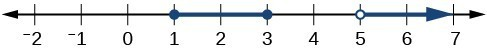### Try It

Given the graph below, specify the graphed set in

1. words
2. set-builder notation
3. interval notation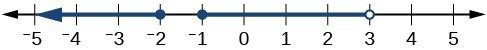The table below gives a summary of interval notation.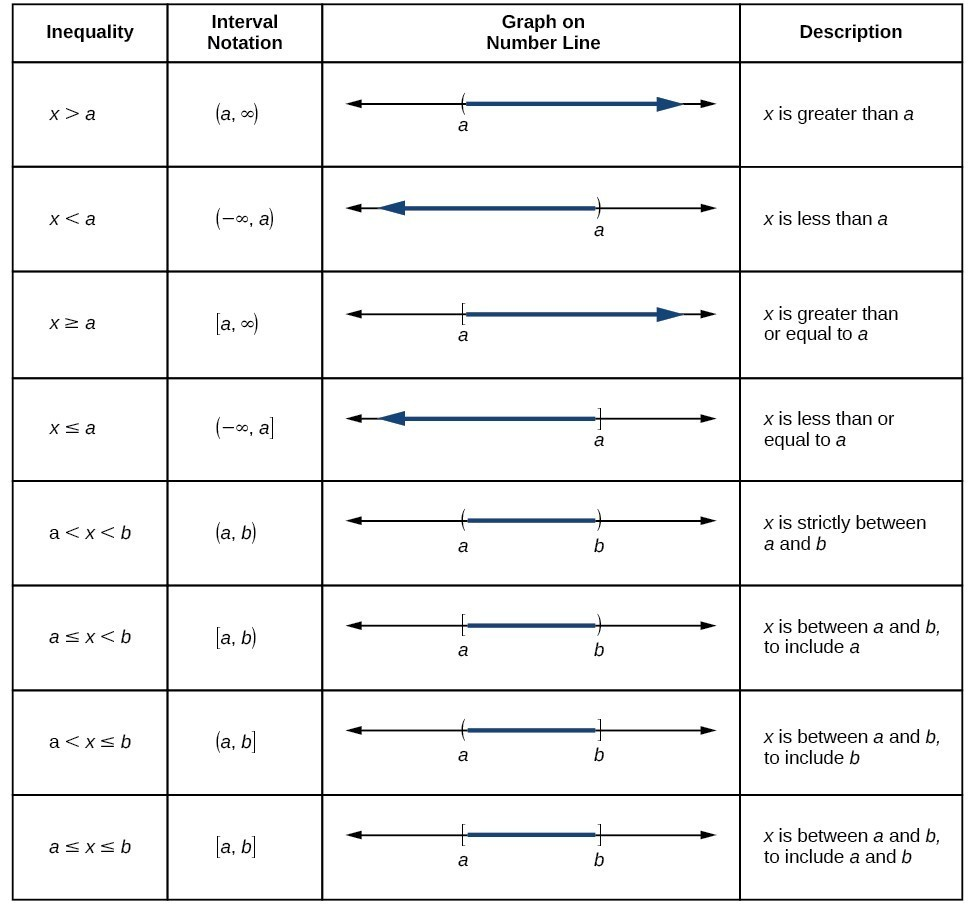## Write Domain and Range Given an Equation

In Functions and Function Notation, we were introduced to the concepts of domain and range. In this section we will practice determining domains and ranges for specific functions. Keep in mind that, in determining domains and ranges, we need to consider what is physically possible or meaningful in real-world examples, such as tickets sales and year in the horror movie example above. We also need to consider what is mathematically permitted. For example, we cannot include any input value that leads us to take an even root of a negative number if the domain and range consist of real numbers. Or in a function expressed as a formula, we cannot include any input value in the domain that would lead us to divide by 0.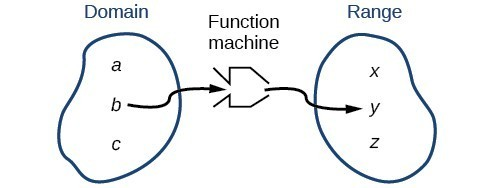We can visualize the domain as a “holding area” that contains “raw materials” for a “function machine” and the range as another “holding area” for the machine’s products.

### Example: Working with a Piecewise Function

A cell phone company uses the function below to determine the cost, $C$, in dollars for $g$ gigabytes of data transfer.

C\left(g\right)=\begin{cases}\begin{align}{25} \hspace{2mm}&\text{ if }\hspace{2mm}{ 0 }<{ g }<{ 2 }\\ { 25+10 }\left(g - 2\right) \hspace{2mm}&\text{ if }\hspace{2mm}{ g}\ge{ 2 }\end{align}\end{cases}

Find the cost of using 1.5 gigabytes of data and the cost of using 4 gigabytes of data.

### How To: Given a piecewise function, sketch a graph.

1. Indicate on the $x$-axis the boundaries defined by the intervals on each piece of the domain.
2. For each piece of the domain, graph on that interval using the corresponding equation pertaining to that piece. Do not graph two functions over one interval because it would violate the criteria of a function.

### Example: Graphing a Piecewise Function

Sketch a graph of the function.

f\left(x\right)=\begin{cases}\begin{align}{ x }^{2} \hspace{2mm}&\text{ if }\hspace{2mm}{ x }\le{ 1 }\\ { 3 } \hspace{2mm}&\text{ if }\hspace{2mm} { 1 }<{ x }\le 2\\ { x } \hspace{2mm}&\text{ if }\hspace{2mm}{ x }>{ 2 }\end{align}\end{cases}

### Try It

Graph the following piecewise function.

f\left(x\right)=\begin{cases}\begin{align}{ x}^{3} \hspace{2mm}&\text{ if }\hspace{2mm}{ x }<{-1 }\\ { -2 } \hspace{2mm}&\text{ if } \hspace{2mm}{ -1 }<{ x }<{ 4 }\\ \sqrt{x} \hspace{2mm}&\text{ if }\hspace{2mm}{ x }>{ 4 }\end{align}\end{cases}

### Try It

You can use an online graphing tool to graph piecewise defined functions. Watch this tutorial video to learn how.

Graph the following piecewise function with an online graphing tool.

f\left(x\right)=\begin{cases}\begin{align}{ x}^{3} \hspace{2mm}&\text{ if }\hspace{2mm}{ x }<{-1 }\\ { -2 } \hspace{2mm}&\text{ if } \hspace{2mm}{ -1 }<{ x }<{ 4 }\\ \sqrt{x} \hspace{2mm}&\text{ if }\hspace{2mm}{ x }>{ 4 }\end{align}\end{cases}

### Q&A

Can more than one formula from a piecewise function be applied to a value in the domain?

No. Each value corresponds to one equation in a piecewise formula.

## Key Concepts

• The domain of a function includes all real input values that would not cause us to attempt an undefined mathematical operation, such as dividing by zero or taking the square root of a negative number.
• The domain of a function can be determined by listing the input values of a set of ordered pairs.
• The domain of a function can also be determined by identifying the input values of a function written as an equation.
• Interval values represented on a number line can be described using inequality notation, set-builder notation, and interval notation.
• For many functions, the domain and range can be determined from a graph.
• An understanding of toolkit functions can be used to find the domain and range of related functions.
• A piecewise function is described by more than one formula.
• A piecewise function can be graphed using each algebraic formula on its assigned subdomain.

## Glossary

interval notation
a method of describing a set that includes all numbers between a lower limit and an upper limit; the lower and upper values are listed between brackets or parentheses, a square bracket indicating inclusion in the set, and a parenthesis indicating exclusion
piecewise function
a function in which more than one formula is used to define the output
set-builder notation
a method of describing a set by a rule that all of its members obey; it takes the form $\left\{x|\text{statement about }x\right\}$